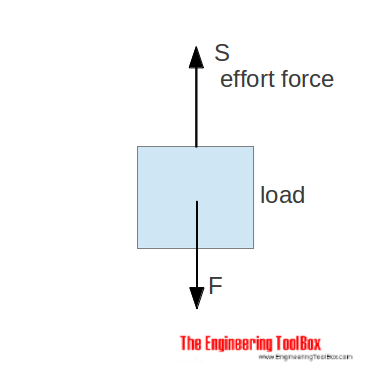Engineering ToolBox - Resources, Tools and Basic Information for Engineering and Design of Technical Applications!

# Force Ratio

## The force ratio is the load force versus the effort force.

A machine is a device that can change the magnitude and line of the action of force.Examples of machines are

A simple machine amplifies the input (effort) force to a larger output (load) force. The force ratio can be expressed as

Fr = F / S                             (1)

where

Fr = force ratio - mechanical advantage

F = load force (N, lbf)

S = effort force (N, lbf)

### Example - Force Ratio for Pulley

The effort force for a pulley with 4 ropes, no friction loss (μ = 1) and load 100 kg is calculated to 245 N.

The force ratio can be estimated to

Fr = (100 kg) (9.81 m/s2) / (245 N)

= 4

## Related Topics

• Mechanics - Forces, acceleration, displacement, vectors, motion, momentum, energy of objects and more.

## Engineering ToolBox - SketchUp Extension - Online 3D modeling!

Add standard and customized parametric components - like flange beams, lumbers, piping, stairs and more - to your Sketchup model with the Engineering ToolBox - SketchUp Extension - enabled for use with the amazing, fun and free SketchUp Make and SketchUp Pro .Add the Engineering ToolBox extension to your SketchUp from the SketchUp Pro Sketchup Extension Warehouse!

Translate

## Privacy

We don't collect information from our users. Only emails and answers are saved in our archive. Cookies are only used in the browser to improve user experience.

Some of our calculators and applications let you save application data to your local computer. These applications will - due to browser restrictions - send data between your browser and our server. We don't save this data.

## Citation

• Engineering ToolBox, (2008). Force Ratio. [online] Available at: https://www.engineeringtoolbox.com/force-ratio-d_1294.html [Accessed Day Mo. Year].

Modify access date.

. .

#### Scientific Online Calculator3 10